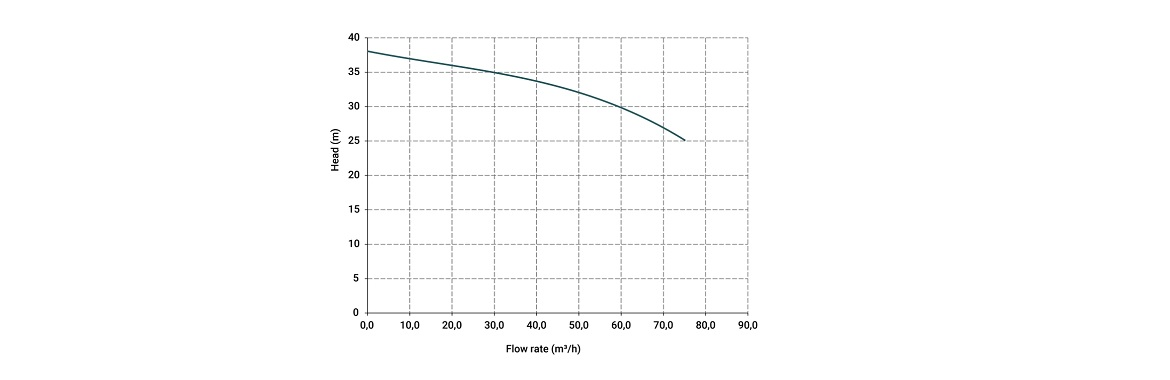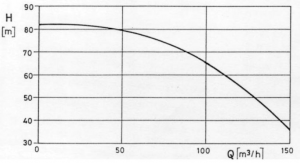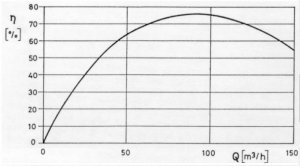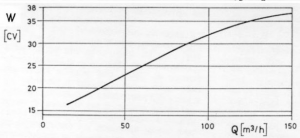# CHARACTERISTIC CURVE OF A CENTRIFUGAL PUMPThe characteristic curve of a pump is the interaction of two variables that describe its performance:

• HEAD: the energy by unit of mass that the pump can supply to the fluid
• FLOW RATE: the quantity of fluid that goes through a section in a certain period of time

See below for an example.The head is plotted on the vertical axis and the flow rate on the horizontal axis.
Two more curves can be plotted, such as the performance curve:which shows the relation between the rated power of the pump and the absorbed power, in relation to the volumetric flow rate and the absorbed power curve:that shows the electric power used in relation to the flow rate.

It must be noted that the centrifugal pump is a mechanical element that is driven by an electric motor, and every characteristic curve refers to a precise rotation of the motor which depends, in the most common asynchronous motors, on the power supply frequency and the number of poles of the motor (e.g. 50 hertz, 2-pole motor: 2900 RPM).

On certain models of centrifugal pumps we can find indications of the NPSH (Net Positive Suction Head), that is the maximum suction head allowed, before triggering cavitations that can damage the mechanical parts of the pump.